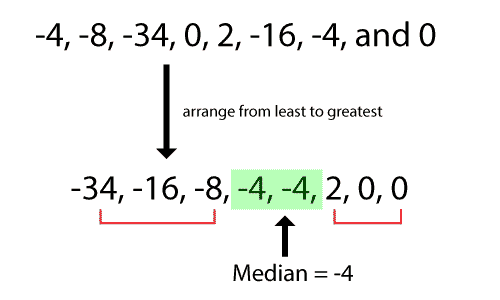# 5 Number Summary Calculator

Enter Information

## How to use five-number summary calculator?

To use this 5 number summary calculator, follow the below steps:

• Enter your values in the given input box.
• Separate each number using a comma.
• Press the Calculate button to see the five-number summary of entered numbers.

The 5 number summary calculator also produces a graph based on the entered values by the user. Hover over the graph to see how your values are distributed on the graph.

The 5 number summary calculator is used to calculate the five-number summary, which consists of five different calculations. In addition to the basic five calculations, it also gives IQR, graph, and descending order.

## What is a Five-number summary?

This summary includes 5 calculations that give information about the range, location, and spread of the data. These calculations include:

• Minimum number
• The first quartile Q1
• Median
• The second quartile Q2, and
• Maximum number

However, if you want to calculate quartiles, you can use our quartile calculator anytime.

## What are the steps to find 5 number summary?

As we have described earlier, this summary consists of a total of five values, which are minimum and maximum numbers, median, and first and third quartile. Follow these steps to calculate the five-number summary.

1. Arrange your data in ascending order (from lower to higher).
2. Get the minimum and maximum values from the arranged values.
3. Find the median from these values. Median can be found by locating the middle value in an arranged set of data. If there are two middle numbers, add them and divide them with 2 to get the median.4. Wrap the parenthesis around the values which are on the left and right side of the median. See the below image.5. Find the first and third quartiles. 1st quartile can be found as the median in the lower quartile, and 3rd quartile can be found as the median in the upper quartile.
6. Write down all values together to get a summary.

### Example:

Let’s calculate 5 number summary using an example to understand the process properly.

Calculate the five number summary in data set 5, 2, 19, 6, 7, 1, 18, 9, 12, 15, 27.

Solution:

Step 1: Arrange the data set in ascending order.

1, 2, 5, 6, 7, 9, 12, 15, 18, 19, 27

Step 2: Get the minimum and maximum values in the data set.

Minimum value = 1

Maximum value = 27

Step 3: Find the median from these values.

Median = 9

Step 4: Wrap the parenthesis around the values which are on the left and right sides of the median.

(1, 2, 5, 6, 7), 9, (12, 15, 18, 19, 27)

Step 5: Find the first and third quartiles. 1st quartile can be found as the median in the lower quartile, and 3rd quartile can be found as the median in the upper quartile.

Q1 = 5

Q2 = 18

Step 6: Write down all values together to get a summary.

1. Minimum = 1
2. Q1 = 5
3. Median = 9
4. Q3 = 18
5. Maximum = 27
Other Languages
User Ratings
• Total Reviews 0
• Overall Rating 0/5
• Stars
Reviews
No Review Yet